Next: Elastic anisotropy with isotropic Up: Materials Previous: The Cailletaud single crystal   Contents

### Elastic anisotropy with isotropic viscoplasticity.

This model describes small deformations for elastically anisotropic materials with a von Mises type yield surface. Often, this model is used as a compromise for anisotropic materials with lack of data or detailed knowledge about the anisotropic behavior in the viscoplastic range.

The total strain is supposed to be the sum of the elastic strain and the plastic strain: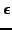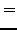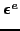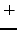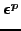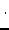(283)

An isotropic hardening variable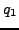and a kinematic hardening tensor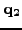are introduced representing the isotropic and kinematic change of the yield surface, respectively. The yield surface takes the form: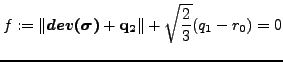(284)

where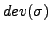is the deviatoric stress tensor, and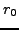is the size of the elastic range at zero yield. The constitutive equations for the hardening variables satisfy: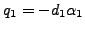(285)

and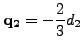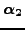(286)

where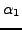and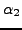are the hardening variables in strain space. It can be shown that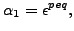(287)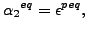(288)

where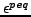is the equivalent plastic strain defined by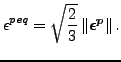(289)

and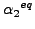is the equivalent value of the tensordefined in a similar way. Thus, the constitutive equations amount to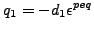(290)

and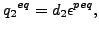(291)

where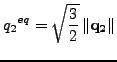(292)

has the meaning of an equivalent stress value or von Mises value. The same applies to. Consequently, the constitutive equations assume a linear relationship between the hardening stress and the equivalent plastic strain.

The constitutive equation for the stress is Hooke's law: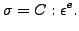(293)

The evolution equations for the plastic strain and the hardening variables in strain space are given by: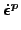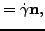(294)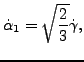(295)

and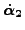(296)

where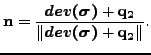(297)

The variable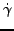is the consistency coefficient known from the Kuhn-Tucker conditions in optimization theory . It can be proven to satisfy: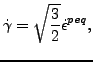(298)

Finally, the creep rate is modeled as a power law function of the yield exceedance and total time t: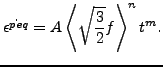(299)

The brackets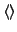reduce negative function values to zero while leaving positive values unchanged, i.e.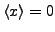if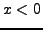and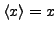if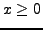.

In the present implementation orthotropic elastic behavior is assumed. Consequently, for each temperature 15 constants need to be defined: the elastic constants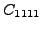,,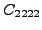,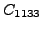,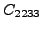,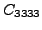,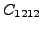,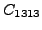,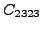, and the viscoplastic constants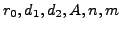.

The material definition consists of a *MATERIAL card defining the name of the material. This name HAS TO START WITH ''ANISO_PLAS'' but can be up to 80 characters long. Thus, the last 70 characters can be freely chosen by the user. Within the material definition a *USER MATERIAL card has to be used satisfying:

First line:

• *USER MATERIAL
• Enter the CONSTANTS parameter and its value, i.e. 15.

Following lines, in sets of 2:

First line of set:

•.
•.
•.
•.
•.
•.
•.
•.
Second line of set:
•.
•.
•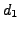.
•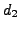.
•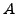.
•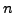.
•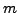.
• Temperature.

Repeat this set if needed to define complete temperature dependence.

The principal axes of the material are assumed to coincide with the global coordinate system. If this is not the case, use an *ORIENTATION card to define a local system.

For this model, there are 14 internal state variables:

• the equivalent plastic strain(1)
• the plastic strain tensor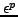(6)
• the isotropic hardening variable(1)
• the kinematic hardening tensor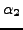(6)

These variables are accessible through the *EL PRINT (.dat file) and *EL FILE (.frd file) keywords in exactly this order (label SDV). The *DEPVAR card must be included in the material definition with a value of 14.

Example:

*MATERIAL,NAME=ANISO_PLAS
*USER MATERIAL,CONSTANTS=15
500000.,157200.,500000.,157200.,157200.,500000.,126200.,126200.,
126200.,0.,0.,0.,1.E-10,5,0.
*DEPVAR
14


defines a single crystal with elastic constants 500000., 157200., 500000., 157200., 157200., 500000., 126200., 126200., 126200., and viscoplastic parameters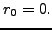,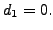,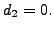,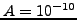,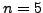and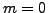. Thus, the yield surface has a zero radius and there is no hardening. Only creep is activated.Next: Elastic anisotropy with isotropic Up: Materials Previous: The Cailletaud single crystal   Contents
guido dhondt 2018-12-15